## Summary and Analysis of Extension Program Evaluation in R

Salvatore S. Mangiafico

### Packages used in this chapter

The packages used in this chapter include:

•  robustbase

•  psych

•  minpack.lm

•  car

•  rcompanion

The following commands will install these packages if they are not already installed:

if(!require(robustbase)){install.packages("robustbase")}
if(!require(pysch)){install.packages("pysch")}
if(!require(nlstools)){install.packages("nlstools")}
if(!require(minpack.lm)){install.packages("minpack.lm")}
if(!require(car)){install.packages("car")}
if(!require(rcompanion)){install.packages("rcompanion")}

### More complex experimental designs

Except for t-tests, the approach of this book for parametric statistics has been to develop linear models (with the lm function) or mixed effects models (with the nlme or lme4 packages) and then to apply analysis of variance, model checking, and post-hoc testing.

These relatively simple models can be expanded to include more independent variables of either continuous or factor type.  Likewise, more complex designs can include nested and crossed factors.  Some traditional experimental designs include Latin square, split plot, and incomplete block.

### Analysis of co-variance.

Analysis of covariance combines continuous independent variables with factor independent variables.

A related design is the paired watershed design which, while it was developed for watershed studies, can be adapted to various situations in a variety of fields.

Analysis of Covariance in Mangiafico, S.S. 2015. An R Companion for the Handbook of Biological Statistics, version 1.09. rcompanion.org/rcompanion/e_04.html.

Clausen, J.C. and J. Spooner. 1993. Paired Watershed Study Design. 841-F-93-009. United States Environmental Protection Agency, Office of Water. Washington, DC. nepis.epa.gov/Exe/ZyPURL.cgi?Dockey=20004PR6.TXT.

Dressing, S.A. and D.W. Meals. 2005. Designing water quality monitoring programs for watershed projects. U.S. Environmental Protection Agency. Fairfax, VA. www.epa.gov/sites/default/files/2015-10/documents/technote2_wq_monitoring.pdf.

### Nonlinear regression and curvilinear regression

Nonlinear and curvilinear regression are similar to linear regression, except that the relationship between the dependent variable and the independent variable is curved in some way.  Specific methods include polynomial regression, spline regression, and nonlinear regression.

Polynomial regression was covered briefly in the previous chapter, while some examples of curvilinear regression are shown below in the “Linear plateau and quadratic plateau models” section in this chapter.

For further discussion, see:

Curvilinear Regression in Mangiafico, S.S. 2015. An R Companion for the Handbook of Biological Statistics, version 1.09. rcompanion.org/rcompanion/e_03.html.

### Multiple regression

Multiple regression is similar to linear regression, except that the model contains multiple independent variables.

The polynomial regression example in the previous chapter is one example of multiple regression.

For further discussion, see:

Multiple Regression in Mangiafico, S.S. 2015. An R Companion for the Handbook of Biological Statistics, version 1.09. rcompanion.org/rcompanion/e_05.html.

### Logistic regression

Logistic regression is similar to linear regression, except that the dependent variable is categorical.  In standard logistic regression, the dependent variable has only two levels, and in multinomial logistic regression, the dependent variable can have more than two levels.

For examples of logistic regression, see the chapter Models for Nominal Data in this book.

### Analysis of count data

When the dependent variable is a counted quantity and not a measured quantity, it is appropriate to use Poisson regression or related techniques.

For a discussion of when standard parametric approaches may or may not be used with count data, see the “Count data may not be appropriate for common parametric tests” section in the Introduction to Parametric Tests chapter.

For examples of appropriate ways to model count data, see the Regression for Count Data chapter in this book.

### Robust techniques

Standard parametric analyses can be sensitive to outliers and other deviations from assumptions of the analyses.  The example below shows that a single point can affect the predicted line from standard linear regression.  It is said that this point has high leverage, as so has high influence in the model.

One approach in these cases is to use nonparametric techniques.

Another approach is to adjust the model to better fit the data.

A third way is to determine if certain data points are true outliers, and then give them further examination.

A fourth way is to use a robust technique such as robust regression.  The references below discuss robust regression and robust techniques appropriate for designs lending themselves to an analysis of variance approach.

One-way Analysis with Permutation Test in Mangiafico, S.S. 2015. An R Companion for the Handbook of Biological Statistics, version 1.09. rcompanion.org/rcompanion/d_06a.html.

Two-way Anova with Robust Estimation in Mangiafico, S.S. 2015. An R Companion for the Handbook of Biological Statistics, version 1.09. rcompanion.org/rcompanion/d_08a.html.

Search for Robust regression in the following:

Correlation and Linear Regression in Mangiafico, S.S. 2015. An R Companion for the Handbook of Biological Statistics, version 1.09. rcompanion.org/rcompanion/e_01.html.

#### Example of robust linear regression

Note that this example does not include checking assumptions of the model, but is intended only to compare the results of ordinary linear regression and robust regression when there is a data point with high influence.

Input=("
X    Y
0    0
1    2
2    5
3    6
4    9
5   10
6   11
7   14
8   16
9   20
10  44
")

##### Linear regression

model = lm(Y ~ X,
data = Data)

summary(model)

Coefficients:
Estimate Std. Error t value Pr(>|t|)
(Intercept)  -3.1364     3.6614  -0.857 0.413888
X             3.1182     0.6189   5.038 0.000701 ***

Residual standard error: 6.491 on 9 degrees of freedom
Multiple R-squared:  0.7383,  Adjusted R-squared:  0.7092
F-statistic: 25.39 on 1 and 9 DF,  p-value: 0.0007013

###### Calculate statistics and plot

Pvalue = pf(summary(model)\$fstatistic,
summary(model)\$fstatistic,
summary(model)\$fstatistic,
lower.tail = FALSE)

R2     = summary(model)\$r.squared

t1     = paste0("p-value: ", signif(Pvalue, digits=3))
t2     = paste0("R-squared: ", signif(R2, digits=3))
t3     = paste0("Intercept: ", signif(coef(model), digits=3))
t4     = paste0("Slope: ", signif(coef(model), digits=3))

plot(Y ~ X,
data = Data,
pch  = 16)

abline(model,
col="blue",
lwd=2)

text(0, 38, labels = t1, pos=4)
text(0, 33, labels = t2, pos=4)
text(0, 28, labels = t3, pos=4)
text(0, 23, labels = t4, pos=4)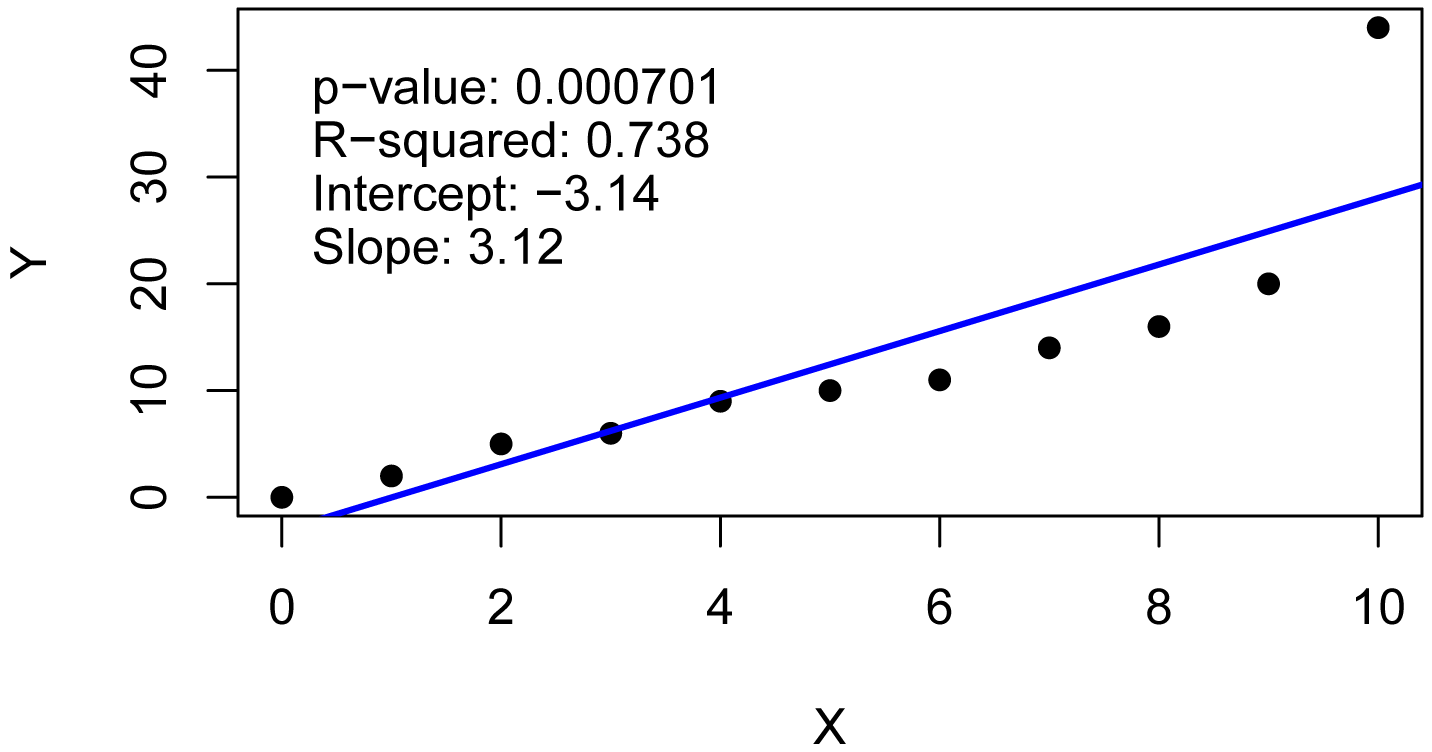###### Cook’s distance

Cook’s distance is a measure of influence of each data point, specifically determining how much predicted values would change if the observation were deleted.  It is sometimes recommended that a Cook’s distance of 1 or more merits further examination of the data point.  However, there are other recommendations for critical values for Cook’s distance.

In this case, the high Cook’s distance for observation 11 makes it a candidate for further evaluation.

barplot(cooks.distance(model))###### Plot of residuals

This plot suggests that the residuals are not independent of the fitted values.  We would probably want to adjust the model by adding additional terms, using a nonlinear or curvilinear approach, or using a robust analysis to minimize the influence of certain data points.

plot(fitted(model),
residuals(model))##### Robust regression

library(robustbase)

model.r = lmrob(Y ~ X,
data = Data)

summary(model.r)

Coefficients:
Estimate Std. Error t value Pr(>|t|)
(Intercept)  0.09015    0.32804   0.275     0.79
X            2.04249    0.10970  18.619  1.7e-08 ***

Robust residual standard error: 0.9378
Multiple R-squared:  0.9817,  Adjusted R-squared:  0.9796

###### Calculate statistics and plot

model.null = lmrob(Y ~ 1,
data = Data)

Pvalue = anova(model.r, model.null)[2,1]

R2     = summary(model.r)\$r.squared

t1     = paste0("p-value: ", signif(Pvalue, digits=3))
t2     = paste0("R-squared: ", signif(R2, digits=3))
t3     = paste0("Intercept: ", signif(coefficients(model.r), digits=3))
t4     = paste0("Slope: ", signif(coefficients(model.r), digits=3))

plot(Y ~ X,
data = Data,
pch  = 16)
abline(model.r,
col="blue",
lwd=2)
text(0, 38, labels = t1, pos=4)
text(0, 33, labels = t2, pos=4)
text(0, 28, labels = t3, pos=4)
text(0, 23, labels = t4, pos=4)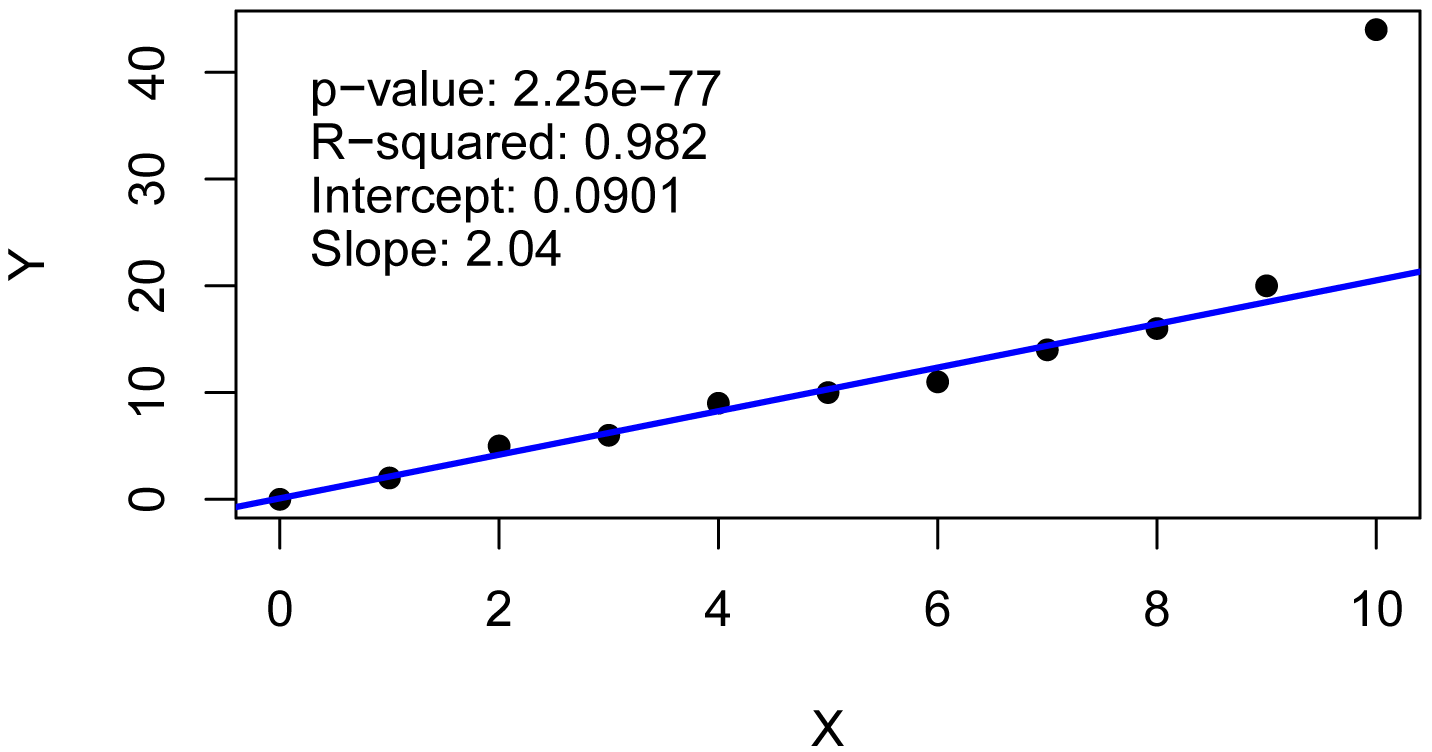### Kendall Theil nonparametric linear regression

Kendall–Theil regression is a completely nonparametric approach to linear regression.  It is robust to outliers in the y values.  It simply computes all the lines between each pair of points, and uses the median of the slopes of these lines.  This method is sometimes called Theil–Sen.  A modified, and preferred, method is named after Siegel.

The mblm function in the mblm package uses the Siegel method by default.  The Theil–Sen procedure can be chosen with the repeated=FALSE option. See library(mblm); ?mblm for more details.

Input=("
X    Y
0    0
1    2
2    5
3    6
4    9
5   10
6   11
7   14
8   16
9   20
10  44
")

library(mblm)

model = mblm(Y ~ X,
data=Data)

summary(model)

Coefficients:
(Intercept)        0   0       6  0.78741
X                  2   0      66  0.00327 **

Pvalue    = as.numeric(summary(model)\$coefficients[2,4])
Intercept = as.numeric(summary(model)\$coefficients[1,1])
Slope     = as.numeric(summary(model)\$coefficients[2,1])
R2        = NULL

t1     = paste0("p-value: ", signif(Pvalue, digits=3))
t2     = paste0("R-squared: ", "NULL")
t3     = paste0("Intercept: ", signif(Intercept, digits=3))
t4     = paste0("Slope: ", signif(Slope, digits=3))

plot(Y ~ X,
data = Data,
pch  = 16)

abline(model,
col="blue",
lwd=2)

text(1, 40, labels = t1, pos=4)
text(1, 36, labels = t2, pos=4)
text(1, 32, labels = t3, pos=4)
text(1, 28, labels = t4, pos=4)### Contrasts in Linear Models

Contrasts can be used in linear models to compare groups of treatments to one another.  For example, if you were testing four curricula and wanted to compare the effect of One and Two vs. Three and Four, you could accomplish this with a single-degree-of freedom contrast.

Contrasts in Linear Models in Mangiafico, S.S. 2015. An R Companion for the Handbook of Biological Statistics, version 1.09. rcompanion.org/rcompanion/h_01.html.

### Linear plateau and quadratic plateau models

Linear plateau and quadratic plateau models are segmented models for bivariate data, typically where the y-value increases with an increase in x to some critical value, beyond which the y-value ceases to increase.  The statistics of interest include the critical value (the x-value above which there is no further increase in y), and the plateau value (the statistically highest value that y reaches).

For another example of these models, see the “Use the best model” section of the Basic Plots chapter.

Also included in this section is an example of curvilinear regression, with a Mitscherlich–Bray model fit to the same data.

#### Examples of linear plateau and quadratic plateau models

Input = ("
Instructor       Grade   Weight  Calories Sodium  Score
'Brendon Small'     6      43     2069    1287      77
'Brendon Small'     6      41     1990    1164      76
'Brendon Small'     6      40     1975    1177      76
'Brendon Small'     6      44     2116    1262      84
'Brendon Small'     6      45     2161    1271      86
'Brendon Small'     6      44     2091    1222      87
'Brendon Small'     6      48     2236    1377      90
'Brendon Small'     6      47     2198    1288      78
'Brendon Small'     6      46     2190    1284      89
'Jason Penopolis'   7      45     2134    1262      76
'Jason Penopolis'   7      45     2128    1281      80
'Jason Penopolis'   7      46     2190    1305      84
'Jason Penopolis'   7      43     2070    1199      68
'Jason Penopolis'   7      48     2266    1368      85
'Jason Penopolis'   7      47     2216    1340      76
'Jason Penopolis'   7      47     2203    1273      69
'Jason Penopolis'   7      43     2040    1277      86
'Jason Penopolis'   7      48     2248    1329      81
'Melissa Robins'    8      48     2265    1361      67
'Melissa Robins'    8      46     2184    1268      68
'Melissa Robins'    8      53     2441    1380      66
'Melissa Robins'    8      48     2234    1386      65
'Melissa Robins'    8      52     2403    1408      70
'Melissa Robins'    8      53     2438    1380      83
'Melissa Robins'    8      52     2360    1378      74
'Melissa Robins'    8      51     2344    1413      65
'Melissa Robins'    8      51     2351    1400      68
'Paula Small'       9      52     2390    1412      78
'Paula Small'       9      54     2470    1422      62
'Paula Small'       9      49     2280    1382      61
'Paula Small'       9      50     2308    1410      72
'Paula Small'       9      55     2505    1410      80
'Paula Small'       9      52     2409    1382      60
'Paula Small'       9      53     2431    1422      70
'Paula Small'       9      56     2523    1388      79
'Paula Small'       9      50     2315    1404      71
'Coach McGuirk'    10      52     2406    1420      68
'Coach McGuirk'    10      58     2699    1405      65
'Coach McGuirk'    10      57     2571    1400      64
'Coach McGuirk'    10      52     2394    1420      69
'Coach McGuirk'    10      55     2518    1379      70
'Coach McGuirk'    10      52     2379    1393      61
'Coach McGuirk'    10      59     2636    1417      70
'Coach McGuirk'    10      54     2465    1414      59
'Coach McGuirk'    10      54     2479    1383      61
")

###  Order factors by the order in data frame
###  Otherwise, R will alphabetize them

Data\$Instructor = factor(Data\$Instructor,
levels=unique(Data\$Instructor))

###  Check the data frame

library(psych)

str(Data)

summary(Data)

### Remove unnecessary objects

rm(Input)

##### Linear plateau model

The nls function in the native stats package can fit nonlinear and curvilinear functions.  To accomplish this, a function—linplat, here—will be defined with the x and y variables (Calories and Sodium) along with parameters (a, b, and clx).  It is the goal of the nls function to find the best fit values for these parameters for these data.

The code below starts with an attempt to find reasonable starting values for the parameters.  If these starting values don’t yield appropriate results, other starting values can be tried.

The fitted parameters a, b, and clx designate the best fit intercept, slope, and critical x value.  The plateau value is calculated as a + b * clx.

###  Find reasonable initial values for parameters

fit.lm    = lm(Sodium ~ Calories, data=Data)

a.ini     = fit.lm\$coefficients
b.ini     = fit.lm\$coefficients
clx.ini   = mean(Data\$Calories)

###  Define linear plateau function

linplat = function(x, a, b, clx)
{ifelse(x < clx, a + b * x,
a + b * clx)}

###  Find best fit parameters

model = nls(Sodium ~ linplat(Calories, a, b, clx),
data = Data,
start = list(a   = a.ini,
b   = b.ini,
clx = clx.ini),
trace = FALSE,
nls.control(maxiter = 1000))

summary(model)

Parameters:
Estimate Std. Error t value Pr(>|t|)
a   -128.85535  116.10819   -1.11    0.273
b      0.65768    0.05343   12.31  1.6e-15 ***
clx 2326.51521   16.82086  138.31  < 2e-16 ***

###### p-value and pseudo R-squared

###  Define null model

nullfunct = function(x, m){m}

m.ini    = mean(Data\$Sodium)

null = nls(Sodium ~ nullfunct(Calories, m),
data = Data,
start = list(m   = m.ini),
trace = FALSE,
nls.control(maxiter = 1000))

###  Find p-value and pseudo R-squared

library(rcompanion)

nagelkerke(model,
null)

\$Pseudo.R.squared.for.model.vs.null
Pseudo.R.squared
Cox and Snell (ML)                   0.892004
Nagelkerke (Cragg and Uhler)         0.892014

\$Likelihood.ratio.test
Df.diff LogLik.diff  Chisq   p.value
-2     -50.077 100.15 1.785e-22

###### Determine confidence intervals for parameter estimates

library(nlstools)

confint2(model,
level = 0.95)

2.5 %       97.5 %
a   -363.1711583  105.4604611
b      0.5498523    0.7654999
clx 2292.5693351 2360.4610904

library(nlstools)

Boot = nlsBoot(model)

summary(Boot)

------
Bootstrap statistics
Estimate   Std. error
a   -143.5189740 111.18292997
b      0.6643267   0.05132983
clx 2326.8124177  16.71482729

------
Median of bootstrap estimates and percentile confidence intervals
Median         2.5%       97.5%
a   -143.5474796 -376.3224461   73.437548
b      0.6638483    0.5652069    0.771089
clx 2327.1279329 2292.5244830 2363.929178

###### Plot data with best fit line

library(rcompanion)

plotPredy(data  = Data,
x     = Calories,
y     = Sodium,
model = model,
xlab  = "Calories per day",
ylab  = "Sodium intake per day")A plot of daily sodium intake vs. daily caloric intake for students.  Best fit linear plateau model is shown.  Critical x value = 2327 calories, and Plateau y = 1401 mg. p < 0.0001.  Pseudo R-squared (Nagelkerke) = 0.892.

###### Plots of residuals

x = residuals(model)

library(rcompanion)

plotNormalHistogram(x)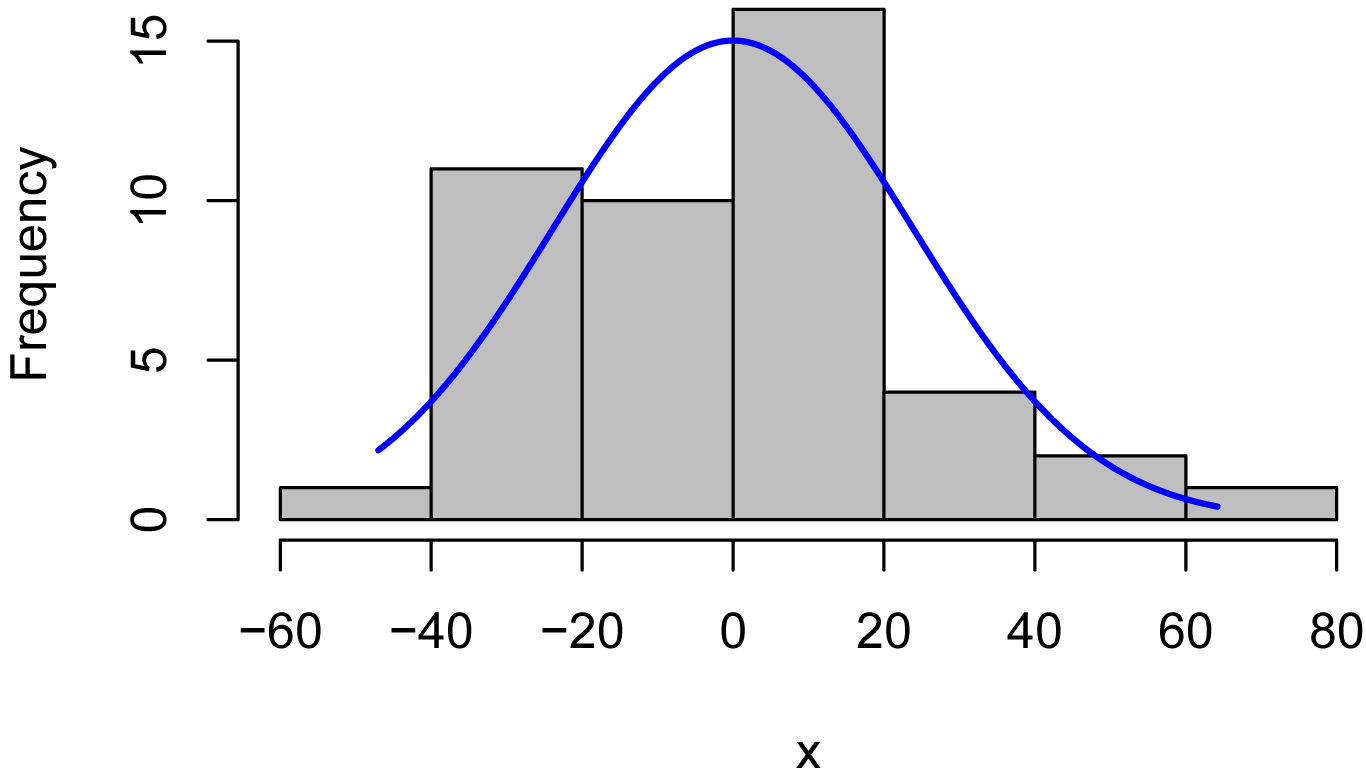plot(fitted(model),
residuals(model))A quadratic plateau model is similar to a linear plateau model, except that the linear segment is replaced with a quadratic function.

The fitted parameters a, b, and clx designate the best fit intercept, linear coefficient, and critical x value.  The quadratic coefficient is calculated as –0.5 * b / clx.  The plateau value is calculated as a + b * clx –0.5 * b * clx.

###  Find reasonable initial values for parameters

fit.lm    = lm(Sodium ~ Calories, data=Data)

a.ini     = fit.lm\$coefficients
b.ini     = fit.lm\$coefficients
clx.ini   = mean(Data\$Calories)

quadplat = function(x, a, b, clx) {
ifelse(x  < clx, a + b * x   + (-0.5*b/clx) * x   * x,
a + b * clx + (-0.5*b/clx) * clx * clx)}

###  Find best fit parameters

model = nls(Sodium ~ quadplat(Calories, a, b, clx),
data = Data,
start = list(a   = a.ini,
b   = b.ini,
clx = clx.ini),
trace = FALSE,
nls.control(maxiter = 1000))

summary(model)

Parameters:
Estimate Std. Error t value Pr(>|t|)
a   -4217.7196   935.1139  -4.510 5.13e-05 ***
b       4.4920     0.8251   5.444 2.49e-06 ***
clx  2504.4059    48.9679  51.144  < 2e-16 ***

###### p-value and pseudo R-squared

###  Define null model

nullfunct = function(x, m){m}

m.ini    = mean(Data\$Sodium)

null = nls(Sodium ~ nullfunct(Calories, m),
data = Data,
start = list(m   = m.ini),
trace = FALSE,
nls.control(maxiter = 1000))

###  Find p-value and pseudo R-squared

library(rcompanion)

nagelkerke(model,
null)

\$Pseudo.R.squared.for.model.vs.null
Pseudo.R.squared
Cox and Snell (ML)                   0.864674
Nagelkerke (Cragg and Uhler)         0.864683

\$Likelihood.ratio.test
Df.diff LogLik.diff  Chisq    p.value
-2     -45.001 90.003 2.8583e-20

###### Determine confidence intervals for parameter estimates

library(nlstools)

confint2(model,
level = 0.95)

2.5 %       97.5 %
a   -6104.855900 -2330.583368
b       2.826818     6.157185
clx  2405.584577  2603.227200

library(nlstools)

Boot = nlsBoot(model)

summary(Boot)

------
Bootstrap statistics
Estimate  Std. error
a   -4294.518746 956.6204011
b       4.560459   0.8448001
clx  2510.154733  53.3134728

------
Median of bootstrap estimates and percentile confidence intervals
Median        2.5%        97.5%
a   -4250.030936 -6311.47965 -2546.971334
b       4.515874     3.03532     6.359601
clx  2504.795426  2425.75206  2626.813217

###### Plot data with best fit line

library(rcompanion)

plotPredy(data  = Data,
x     = Calories,
y     = Sodium,
model = model,
xlab  = "Calories per day",
ylab  = "Sodium intake per day")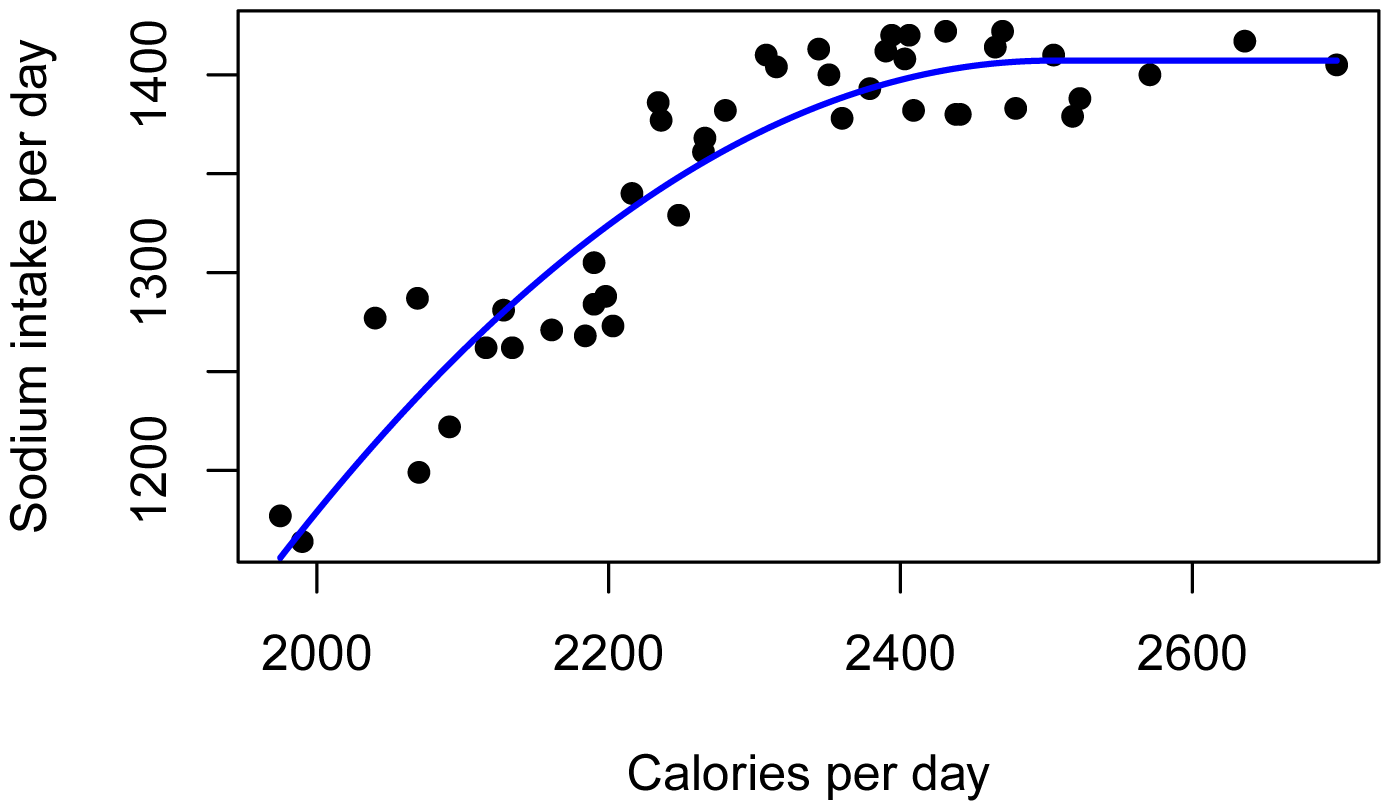A plot of daily sodium intake vs. daily caloric intake for students.  Best fit quadratic plateau model is shown.  Critical x value = 2504 calories, and Plateau y = 1403 mg. p < 0.0001.  Pseudo R-squared (Nagelkerke) = 0.865.

###### Plots of residuals

x = residuals(model)

library(rcompanion)

plotNormalHistogram(x)plot(fitted(model),
residuals(model))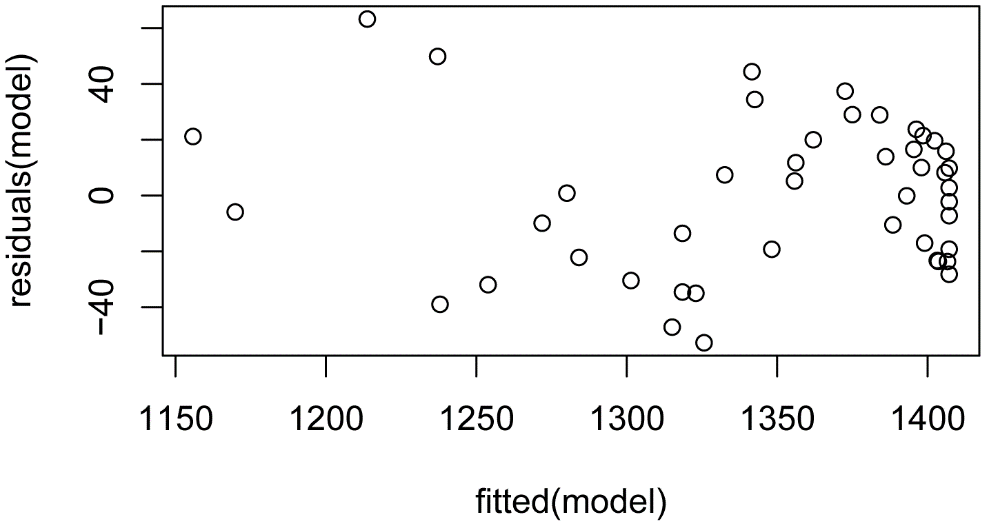##### Mitscherlich–Bray  model

This model is an example of curvilinear regression, specifically with a general exponential model.  The model here is specified as y = abecx.

In the function below, the constant D is set to the minimum of Calories + 1.  This will be subtracted from the x values because the numerically large values for Calories make the fitting of the exponential function difficult without doing this subtraction.

###  Find reasonable initial values for parameters

a.ini   = 1400
b.ini   = -120
c.ini   = 0.01

###  Set a constant to adjust x values

D       = 1974

###  Define exponential function

expon = function(x, a, b, c) {
a + b * exp(-1 * c * (x - D))}

###  Find best fit parameters

model = nls(Sodium ~ expon(Calories, a, b, c),
data = Data,
start = list(a = a.ini,
b = b.ini,
c = c.ini),
trace = FALSE,
nls.control(maxiter = 10000))

summary(model)

Parameters:
Estimate Std. Error t value Pr(>|t|)
a  1.450e+03  2.309e+01  62.790  < 2e-16 ***
b -2.966e+02  2.142e+01 -13.846  < 2e-16 ***
c  3.807e-03  7.973e-04   4.776  2.2e-05 ***

###### p-value and pseudo R-squared

###  Define null model

nullfunct = function(x, m){m}

m.ini    = mean(Data\$Sodium)

null = nls(Sodium ~ nullfunct(Calories, m),
data = Data,
start = list(m   = m.ini),
trace = FALSE,
nls.control(maxiter = 1000))

###  Find p-value and pseudo R-squared

library(rcompanion)

nagelkerke(model,
null)

\$Pseudo.R.squared.for.model.vs.null
Pseudo.R.squared
Cox and Snell (ML)                   0.842908
Nagelkerke (Cragg and Uhler)         0.842918

\$Likelihood.ratio.test
Df.diff LogLik.diff  Chisq    p.value
-2     -41.646 83.292 8.1931e-19

###### Determine confidence intervals for parameter estimates

library(nlstools)

confint2(model,
level = 0.95)

2.5 %        97.5 %
a  1.403060e+03  1.496244e+03
b -3.398171e+02 -2.533587e+02
c  2.198512e-03  5.416355e-03

library(nlstools)

Boot = nlsBoot(model)

summary(Boot)

------
Bootstrap statistics
Estimate   Std. error
a  1.453109e+03 2.522906e+01
b -3.005815e+02 2.244473e+01
c  3.850955e-03 7.832523e-04

------
Median of bootstrap estimates and percentile confidence intervals
Median          2.5%         97.5%
a  1.449234e+03  1.415464e+03  1.513570e+03
b -2.998719e+02 -3.474264e+02 -2.566514e+02
c  3.827519e-03  2.390379e-03  5.410459e-03

###### Plot data with best fit line

library(rcompanion)

plotPredy(data  = Data,
x     = Calories,
y     = Sodium,
model = model,
xlab  = "Calories per day",
ylab  = "Sodium intake per day")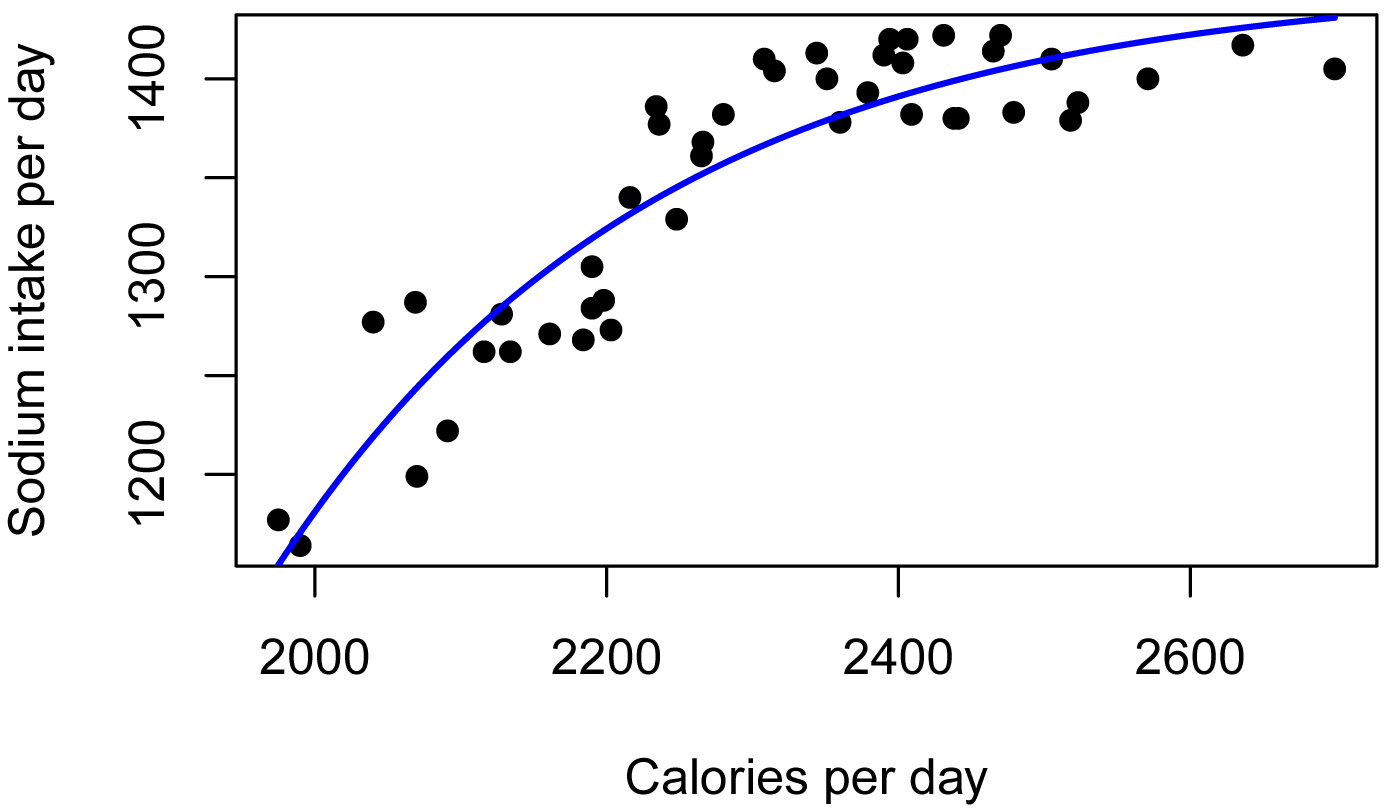A plot of daily sodium intake vs. daily caloric intake for students.  Best fit exponential model is shown.  p < 0.0001.  Pseudo R-squared (Nagelkerke) = 0.843.

###### Plots of residuals

x = residuals(model)

library(rcompanion)

plotNormalHistogram(x)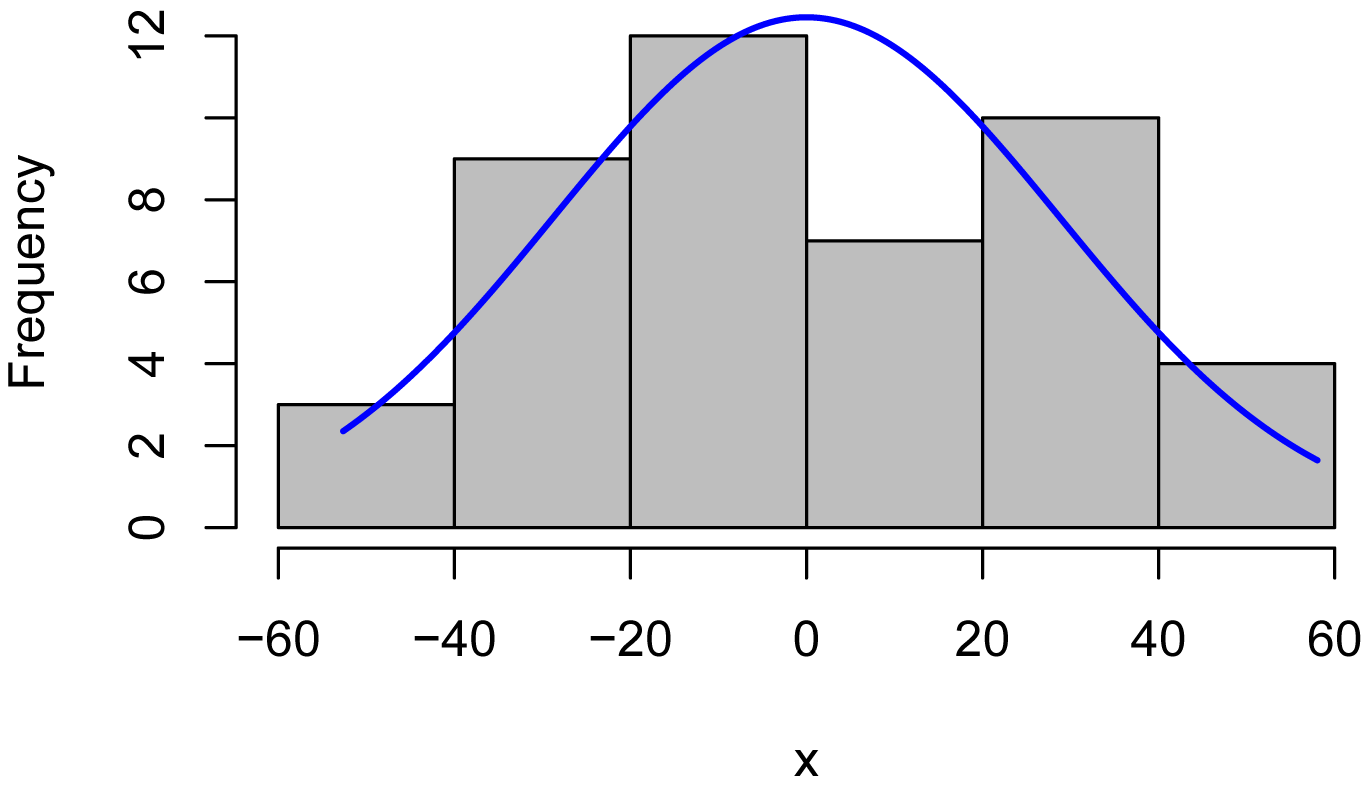plot(fitted(model),
residuals(model))##### Better functions to fit nonlinear regression

Finding reasonable starting values for some functions can be difficult, and the nls function may not find a solution for certain functions if starting values are not close to their best values.  One issue is that the Gauss–Newton method that nls uses will fail in some situations.

Typically, I will put my data into a spreadsheet and make a plot of the function I am trying to fit.  I can then change parameter values and watch the curve change until I get the curve reasonably close to the data.

The package minpack.lm has a function nlsLM, which uses the Levenberg–Marquardt method.  It is more successful at fitting parameters for difficult functions when the initial values for parameters are poor.  An example of using this package is shown in the “Fitting curvilinear models with the minpack.lm package” below.

(The package nlmrt uses the Marquardt–Nash method, which is also tolerant of difficult functions and poor starting values for parameters.  However, at the time of writing, this package is not available for newer versions of R.)

##### Fitting curvilinear models with the minpack.lm package

For a plot of the best fit line and plots of residuals, see the previous section of this chapter.

library(minpack.lm)

###  Define exponential function

expon = function(x, a, b, c) {
a + b * exp(-1 * c * (x - D))}

###  Find best fit parameters

model = nlsLM(Sodium ~ expon(Calories, a, b, c),
data = Data,
start = list(a = 1400,
b = -1,
c = 0.1))

summary(model)

Parameters:
Estimate Std. Error t value Pr(>|t|)
a  1.450e+03  2.309e+01  62.789  < 2e-16 ***
b -2.966e+02  2.142e+01 -13.846  < 2e-16 ***
c  3.807e-03  7.972e-04   4.776  2.2e-05 ***

### Cate–Nelson analysis

Cate–Nelson analysis is a useful approach for some bivariate data that don’t fit neatly into linear model, linear–plateau model, or curvilinear model.  The analysis divides the data into two populations: those x values that are likely to have high y values, and those x values that are likely to have low y values.

For more discussion and examples, see:

Cate–Nelson Analysis in Mangiafico, S.S. 2015. An R Companion for the Handbook of Biological Statistics, version 1.09. rcompanion.org/rcompanion/h_02.html.

library(rcompanion)

cateNelson(x          = Data\$Calories,
y          = Data\$Sodium,
plotit     = TRUE,
hollow     = TRUE,
xlab       = "Calories per day",
ylab       = "Sodium intake, mg per day",
trend      = "positive",
clx        = 1,
cly        = 1,
xthreshold = 0.10,
ythreshold = 0.15)

Final model:

n    CLx       SS  CLy Q.I Q.II Q.III Q.IV  Fisher.p.value
1 45 2209.5 190716.1 1317   0   30     0   15  2.899665e-12A plot of daily sodium intake vs. daily caloric intake for students, with a Cate–Nelson analysis applied.  Students with a Caloric intake greater than 2210 calories tended to have a sodium intake greater than 1317 mg per day.